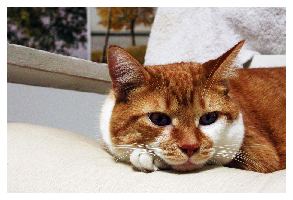## Lesson Video:

This article is also a Jupyter Notebook available to be run from the top down. There will be code snippets that you can then run in any environment.

Below are the versions of fastai, fastcore, and wwf currently running at the time of writing this:

• fastai: 2.1.10
• fastcore: 1.3.13
• wwf: 0.0.7

• Keypoint or Pose detection:
• n keypoints are found using a CNN where n = max number of keypoints present

## Libraries

from fastai.vision.all import *


## Cleaning Some Data:

For our dataset, we will be working from the Kaggle Cats dataset. Now we are purposefully going to go about cleaning our data beforehand so we understand what it is like

url = "https://drive.google.com/uc?id=1ffJr3NrYPqzutcXsYIVNLXzzUaC9RqYM"

!gdown {url}

Downloading...
To: /content/cat-dataset.zip
4.33GB [01:19, 54.2MB/s]


Now that it's downloaded, let's unzip it using ZipFile

from zipfile import ZipFile

with ZipFile('cat-dataset.zip', 'r') as zip_ref:
zip_ref.extractall()


How is the data stored? Let's talk a look by walking on our folders:

import os

[x for x in os.walk('cats')]

['cats',
'cats/CAT_05',
'cats/CAT_06',
'cats/CAT_02',
'cats/CAT_01',
'cats/CAT_03',
'cats/CAT_04',
'cats/CAT_00']

We have some duplicate folders, let's get rid of the CAT_ directory and just work out of the cats folder

for i in range(7):
path = Path(f'CAT_0{i}')
shutil.rmtree(path)


Now we need to move all the files up one level. We can use pathlib

for i in range(7):
paths = Path(f'cats/CAT_0{i}').ls()
for path in paths:
p = Path(path).absolute()
par = p.parents
p.rename(par/p.name)


How is our data labeled? Our keypoints are available via an image's corresponding .cat file. Let's make sure we have an equal number of labels to images

path = Path('cats')

lbls = get_files(path, extensions='.cat')
imgs = get_image_files(path)

test_eq(len(lbls), len(imgs))


We're good to go! - Or are we

## Visualizing our data

Let's first grab a label based on a file name

def img2kpts(f): return f'{str(f)}.cat'


Let's try this out on an image

fname = imgs

img = PILImage.create(fname)

img.show()

<matplotlib.axes._subplots.AxesSubplot at 0x7ff19e44fcf8>Now let's grab some coordinates!

kpts = np.genfromtxt(img2kpts(fname)); kpts

array([  9., 563., 411., 736., 404., 669., 545., 404., 340., 380., 148.,
528., 261., 739., 254., 869., 123., 811., 325.])

Wait, that's not our keypoints. What is this?

It is, go back to the Kaggle and they describe how it is done. The number of points by default are 9 (the first value in our list):

• Left eye
• Right eye
• Mouth
• Left ear 1
• Left ear 2
• Left ear 3
• Right ear 1
• Right ear 2
• Right ear 3

Now we need to seperate our keypoints into pairs and a tensor

def sep_points(coords:array):
"Seperate a set of points to groups"
kpts = []
for i in range(1, int(coords*2), 2):
kpts.append([coords[i], coords[i+1]])
return tensor(kpts)

pnts = sep_points(kpts); pnts

tensor([[563., 411.],
[736., 404.],
[669., 545.],
[404., 340.],
[380., 148.],
[528., 261.],
[739., 254.],
[869., 123.],
[811., 325.]])

Now let's put it all together. We need to return some TensorPoints to have it work in fastai

First let's take what we did above and make a get_y function

def get_y(f:Path):
"Get keypoints for f image"
pts = np.genfromtxt(img2kpts(f))
return sep_points(pts)


Now there is one more bit of cleaning we need to do, and that is to make sure all my points are within the bounds of my image. But how do I do this? Let's write a list of bad_fnames in which we run the following test:

1. Open an image and the points
2. If any point is outside the image, remove the file
3. If any point is negative, remove the file
bad_imgs = []

for name in imgs:
im = PILImage.create(name)
y = get_y(name)
for x in y:
if x < im.size:
if x < 0:
if x < im.size:
if x < 0:
else:
else:


len(bad_imgs)

1430

That's a lot! But couldn't we also get repeats from the above code? Let's check for that

len(set(bad_imgs))

1062

We could. So in total we have 1,062 images who's points go out of bounds. There's a few different ways we can deal with this.

1. Remove said image
2. Zero those points to (-1,-1) (through a transform
3. Keep the points

Each have their benefits. We'll do #1

for name in list(set(bad_imgs)):


Now that we've removed all the bad images, let's continue

imgs = get_image_files(path)
fname = imgs
img = PILImage.create(fname)


Now let's get our TensorPoints, just to show an example

def get_ip(img:PILImage, pts:array): return TensorPoint(pts, sz=img.size)

ip = get_y(fname); ip

tensor([[563., 411.],
[736., 404.],
[669., 545.],
[404., 340.],
[380., 148.],
[528., 261.],
[739., 254.],
[869., 123.],
[811., 325.]])
tp = get_ip(img, ip)


Now we can visualize our points. We can pass in an axis to overlay them on top of our image

ax = img.show(figsize=(12,12))
tp.show(ctx=ax)

<matplotlib.axes._subplots.AxesSubplot at 0x7ff19f70aa90>